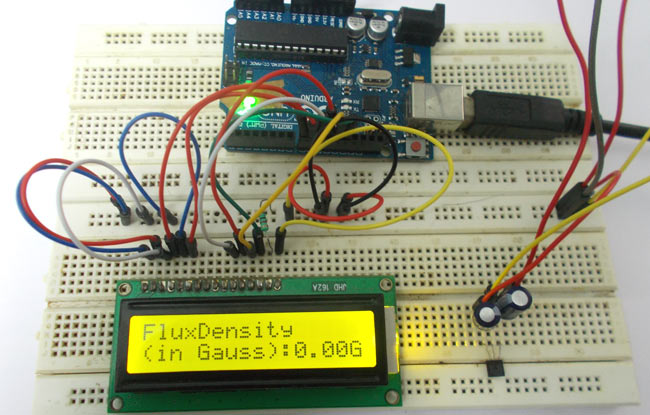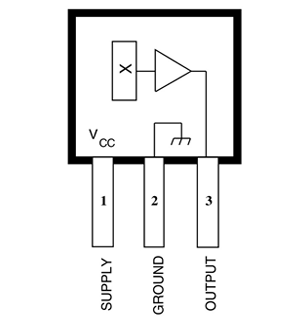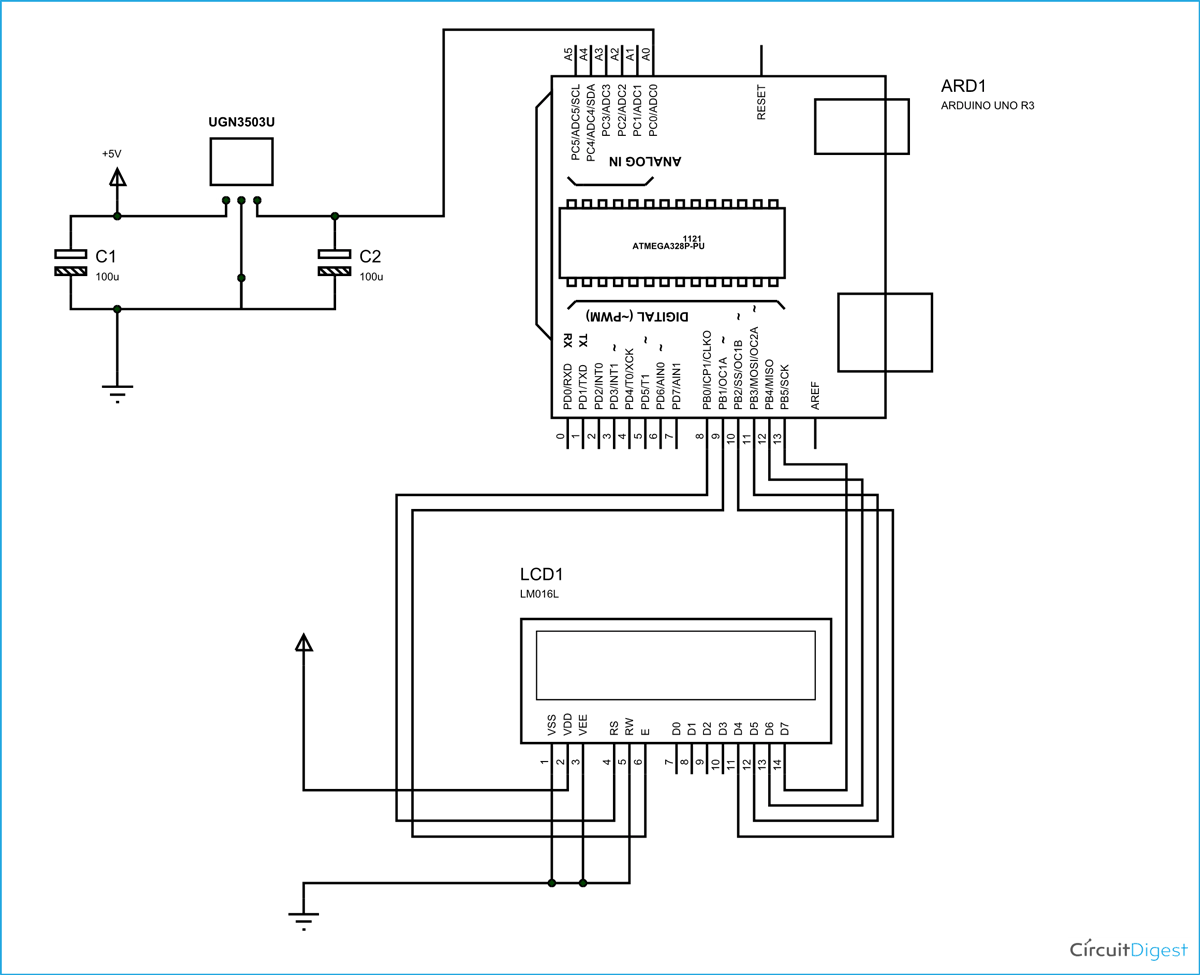# Magnetic Field Strength Measurement using Arduino

In this project we are using the concept of ADC (Analog to Digital Conversion) in ARDUINO UNO. We are going to use a Hall Effect sensor and Arduino uno to measure the field strength of a magnet. The sensor which we have used here is UGN3503U. This is a hall sensor which senses the magnetic field strength and provides a varying voltage at output proportional to the field strength. This sensor picks up field strength in the units of ‘GAUSS’.So with this sensor we will have field strength as varying voltage. By using ADC feature we will convert this voltage to a number. This number represents the field strength and is shown on LCD. [Also check: 16×2 LCD Interfacing with Arduino]Arduino has six ADC channels. In those any one or all of them can be used as inputs for analog voltage. The UNO ADC is of 10 bit resolution (so the integer values from (0-(2^10) 1023)). This means that it will map input voltages between 0 and 5 volts into integer values between 0 and 1023. So for every (5/1024= 4.9mV) per unit.

In all of this we are going to connect a potentiometer or pot to the ‘A0’ channel, and we are going to show the ADC result in a simple display. The simple displays are 16×1 and 16×2 display units. The 16×1 display unit will have 16 characters and are in one line. The 16×2 will have 32 characters in total 16in 1st line and another 16 in 2nd line. Here one must understand that in each character there are 5×10=50 pixels so to display one character all 50 pixels must work together, but we need not have to worry about that because there a another controller (HD44780) in the display unit which does the job of controlling the pixels (you can see it in LCD unit, it is the black eye at the back).

READ  [FTC] Open Feathercoin ATM

### Components Required

Hardware: ARDUINO UNO, power supply (5v), JHD_162ALCD (16x2LCD), 100uF capacitor (2pieces), UGn3503U.

Software: arduino IDE (Arduino nightly)The above figure shows the circuit diagram for magnetic field measurement using arduino uno.

In 16×2 LCD there are 16 pins over all if there is a back light, if there is no back light there will be 14 pins. One can power or leave the back light pins. Now in the 14 pins there are 8 data pins (7-14 or D0-D7), 2 power supply pins (1&2 or VSS&VDD or GND&+5v), 3rd pin for contrast control (VEE-controls how thick the characters should be shown) and 3 control pins (RS&RW&E).

In the circuit above, you can observe I have only took two control pins, the contrast bit and READ/WRITE are not often used so they can be shorted to ground. This puts LCD in highest contrast and read mode. We just need to control ENABLE and RS pins to send characters and data accordingly.

The connections which are done for LCD are given below:

PIN1 or VSS to ground

PIN2 or VDD or VCC to +5v power

PIN3 or VEE to ground (gives maximum contrast best for a beginner)

PIN4 or RS (Register Selection) to PIN8 of ARDUINO UNO

PIN5 or RW (Read/Write) to ground (puts LCD in read mode eases the communication for user)

PIN6 or E (Enable) to PIN9 of ARDUINO UNO

PIN11 or D4 to PIN10 of ARDUINO UNO

PIN12 or D5 to PIN11 of ARDUINO UNO

PIN13 or D6 to PIN12 of ARDUINO UNO

READ  nRF24L01+ with ATtiny85 3 Pins

PIN14 or D7 to PIN13 of ARDUINO UNO

The ARDUINO IDE allows the user to use LCD in 4 bit mode. This type of communication enables the user to decrease the pin usage on ARDUINO, unlike other the ARDUINO need not be programmed separately for using it in 4 it mode because by default the ARDUINO is set up to communicate in 4 bit mode. In the circuit you can see we used 4bit communication (D4-D7). So from mere observation from above table we are connecting 6 pins of LCD to controller in which 4 pins are data pins and 2 pins for control.

#### This Post / Project can also be found using search terms:

• electromagnetic field strength measurement using aurdino
• magnenting field stregth measurement using arduino
• Magnetic field strength meas